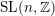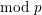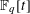Home

### Elementary number theory (11Axx)

• Article
• ##### PRIME-UNIVERSAL QUADRATIC FORMS$ax^{2}+by^{2}+cz^{2}$ AND$ax^{2}+by^{2}+cz^{2}+dw^{2}$
• Bulletin of the Australian Mathematical Society, First View

### Sequences and sets (11Bxx)

• Article
• ##### Equidistribution of Farey sequences on horospheres in covers of$\text{SL}(n+1,\mathbb{Z})\backslash \text{SL}(n+1,\mathbb{R})$ and applications
• Ergodic Theory and Dynamical Systems, First View

### Diophantine equations (11Dxx)

• Article
• ##### Equidistribution of Farey sequences on horospheres in covers of$\text{SL}(n+1,\mathbb{Z})\backslash \text{SL}(n+1,\mathbb{R})$ and applications
• Ergodic Theory and Dynamical Systems, First View

### Forms and linear algebraic groups (11Exx)

• Article
• ##### PRIME-UNIVERSAL QUADRATIC FORMS$ax^{2}+by^{2}+cz^{2}$ AND$ax^{2}+by^{2}+cz^{2}+dw^{2}$
• Bulletin of the Australian Mathematical Society, First View

• Article
• ##### Words have bounded width in$\operatorname{SL}(n,\mathbb{Z})$
• Compositio Mathematica, Volume 155, Issue 7

### Discontinuous groups and automorphic forms (11Fxx)

• Article
• ##### A SHIFTED CONVOLUTION SUM OF$d_{3}$ AND THE FOURIER COEFFICIENTS OF HECKE–MAASS FORMS II
• Bulletin of the Australian Mathematical Society, First View

### Probabilistic theory: distribution modulo $1$; metric theory of algorithms (11Kxx)

• Article
• ##### Equidistribution of Farey sequences on horospheres in covers of$\text{SL}(n+1,\mathbb{Z})\backslash \text{SL}(n+1,\mathbb{R})$ and applications
• Ergodic Theory and Dynamical Systems, First View

### Exponential sums and character sums (11Lxx)

• Article
• ##### One-Level Density of Low-lying Zeros of Quadratic and Quartic Hecke$L$ -functions
• Canadian Journal of Mathematics, First View

### Additive number theory; partitions (11Pxx)

• Article
• ##### ARITHMETIC PROPERTIES OF COEFFICIENTS OF THE MOCK THETA FUNCTION$B(q)$
• Bulletin of the Australian Mathematical Society, First View

### Algebraic number theory: local and $p$-adic fields (11Sxx)

• Article
• ##### REFINED SWAN CONDUCTORS$\text{mod}~p$ OF ONE-DIMENSIONAL GALOIS REPRESENTATIONS
• Nagoya Mathematical Journal, Volume 236
• Article
• ##### LOCALLY ANALYTIC VECTORS AND OVERCONVERGENT$(\unicode[STIX]{x1D711},\unicode[STIX]{x1D70F})$ -MODULES
• Journal of the Institute of Mathematics of Jussieu, First View

### Finite fields and commutative rings (number-theoretic aspects) (11Txx)

• Article
• ##### LINEAR AND QUADRATIC UNIFORMITY OF THE MÖBIUS FUNCTION OVER$\mathbb{F}_{q}[t]$
• Mathematika, Volume 65, Issue 3